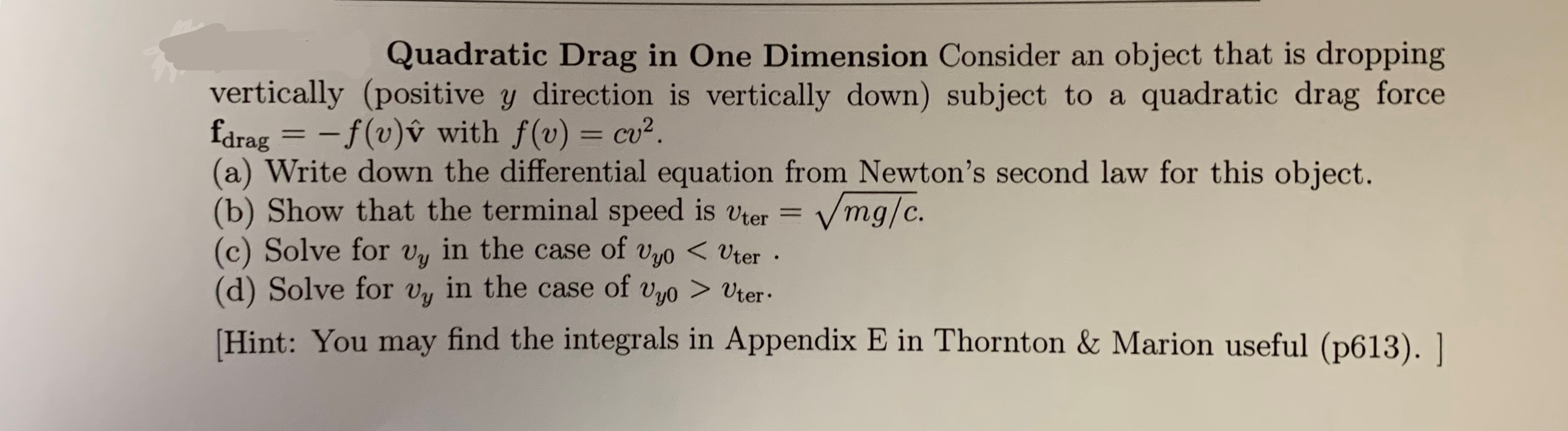# Quadratic Drag in One Dimension Consider an object that is droppingquadratic drag forcevertically (positive y direction is vertically down) subject to afdrag = -f(v)v with f(v) cu2.(a) Write down the differential equation from Newton's second law for this object.(b) Show that the terminal speed is vter =(c) Solve for vy in the case of vyo UterVmg/c.Hint: You may find the integrals in Appendix E in Thornton & Marion useful (p613). |

Question
89 viewshelp_outlineImage TranscriptioncloseQuadratic Drag in One Dimension Consider an object that is dropping quadratic drag force vertically (positive y direction is vertically down) subject to a fdrag = -f(v)v with f(v) cu2. (a) Write down the differential equation from Newton's second law for this object. (b) Show that the terminal speed is vter = (c) Solve for vy in the case of vyo Uter Vmg/c. Hint: You may find the integrals in Appendix E in Thornton & Marion useful (p613). | fullscreen
check_circle

Step 1

(a) Using Newton’s law,

Step 2

(b) The object has zero acceleration when it reaches terminal speed. Therefore,

Step 3

(c) Re-arrange the equation ...

### Want to see the full answer?

See Solution

#### Want to see this answer and more?

Solutions are written by subject experts who are available 24/7. Questions are typically answered within 1 hour.*

See Solution
*Response times may vary by subject and question.
Tagged in

### Physics